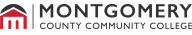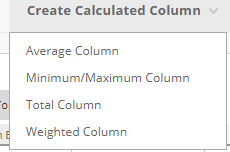# Faculty - Calculated Column Options in the Blackboard Grade Center2018

# Faculty - Calculated Column Options in the Blackboard Grade Center

 There are 4 Calculated Column options to select from in the Grade Center. By default, you are provided a Weighted Total and Total column. You may remove the column that you do not need to use for calculating student grades. If you accidentally remove a Calculated Column, you can always re-add it by going to the Full Grade Center and selecting "Create Calculated Column." This article will provide you with an explanation of each of the Calculated Column options.

 1. Scroll down to Course Management and click Grade Center. Then click Full Grade Center.2. Click Create Calculated Column.3. Determine what kind of Calculated Column you want to add to your Grade Center: Average Column: The Average Column calculates the average for a selected number of columns. For example, you can calculate the average for all tests. The average is calculated by taking the percent earned divided by the number of items selected to calculate the average. Example: Column 1 % + Column 2 % + Column 3 % + Column 4 % = % earned ÷ 4 columns = Average percentage score     Minimum/Maximum Column: The Minimum/Maximum Column calculates the minimum or maximum grade for a selected number of columns. For example, you can find the minimum score for all tests. The percentage value for each column selected in the calculation is compared up to four decimal places and either the lowest or highest value is show.  Example:  Values: 9/10, 4/5, 3/3 Percentage Equivalents: 90.0000%, 80.0000%, 100.0000% Minimum: 80.0000% Maximum: 100.0000%      Total Column: The total column calculates a grade based on the cumulative points earned, related to the points allowed. You can select what columns to calculate. A Total column is created by default. Example: Column 1 points earned + Column 2 points earned + Column 3 points earned + Column 4 point earned = Total points earned out of total points possible     Weighted Column: The Weighted Total column calculates a grade based on selected columns and their categories, and their identified percentages. For example, if Quizzes in your course are worth 15% of a students' overall grade then you would need to use a Weighted Column to calculate grades.  Example: Make sure all assessments in your course are given the correct Category. For example, all Quizzes should have the Category of Quiz associated with it. Since Quizzes are worth 15% of a students' overall grade, you will need to add the Quiz Category to your Weighted Total column. For more specific information please view the following article, Setting Up your Grade Center: Weighted Total Video
1 (1)You've Already Voted.